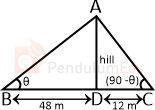Question of The Day07-06-2021

Point A and B are on the opposite side of the hill and were 48 m and 12 m away from the foot of the hill respectively. The angle of elevation from Point A to the peak of the hill is complementary to the angle of elevation from point B to the peak of the hill. What is the height of the hill?

Correct Answer : c ) 24 m

Explanation :

According to the question

Let ∠DBA = θ and AD is the height of the Hill

A and B are on the opposite side of the Hill and were 48 m and 12 m respectively

Angle of elevation to the top of the hill to the point A and B is complementary

We know that two angles are complementary to each other if the sum is 90⁰.

⇒∠DBA = θ , ∠DCA = (90 – θ)We know that

tanθ=$$p \over b$$

Where p is the perpendicular and b is the base of the triangle

In △ABD

tanθ=$$AD \over BD$$

Substituting the values

tanθ=$$AD \over 48$$……..(1)

tan(90-θ)=$$AD \over 12$$

We know that

tan(90 – θ) = cot θ

cotθ=$$1 \over \tan\ θ$$

⇒cotθ=$$AD \over 12$$

⇒tanθ=$$12 \over AD$$…….(2)

comparing equation (1) and (2)

$${AD \over 48}$$=$${12 \over AD}$$

⇒AD=$$\sqrt {576}$$

Hence, (c) is the correct answer.

Such type of question is asked in various government exams like SSC CGL, SSC MTS, SSC CPO, SSC CHSL, RRB JE, RRB NTPC, RRB GROUP D, RRB OFFICER SCALE-I, IBPS PO, IBPS SO, RRB Office Assistant, IBPS Clerk, RBI Assistant, IBPS RRB OFFICER SCALE 2&3, UPSC CDS, UPSC NDA etc.

Read Daily Current Affairs, Banking Awareness, Hindi Current Affairs, Word of the Day, and attempt free mock tests at PendulumEdu and boost your preparation for the actual exam.0